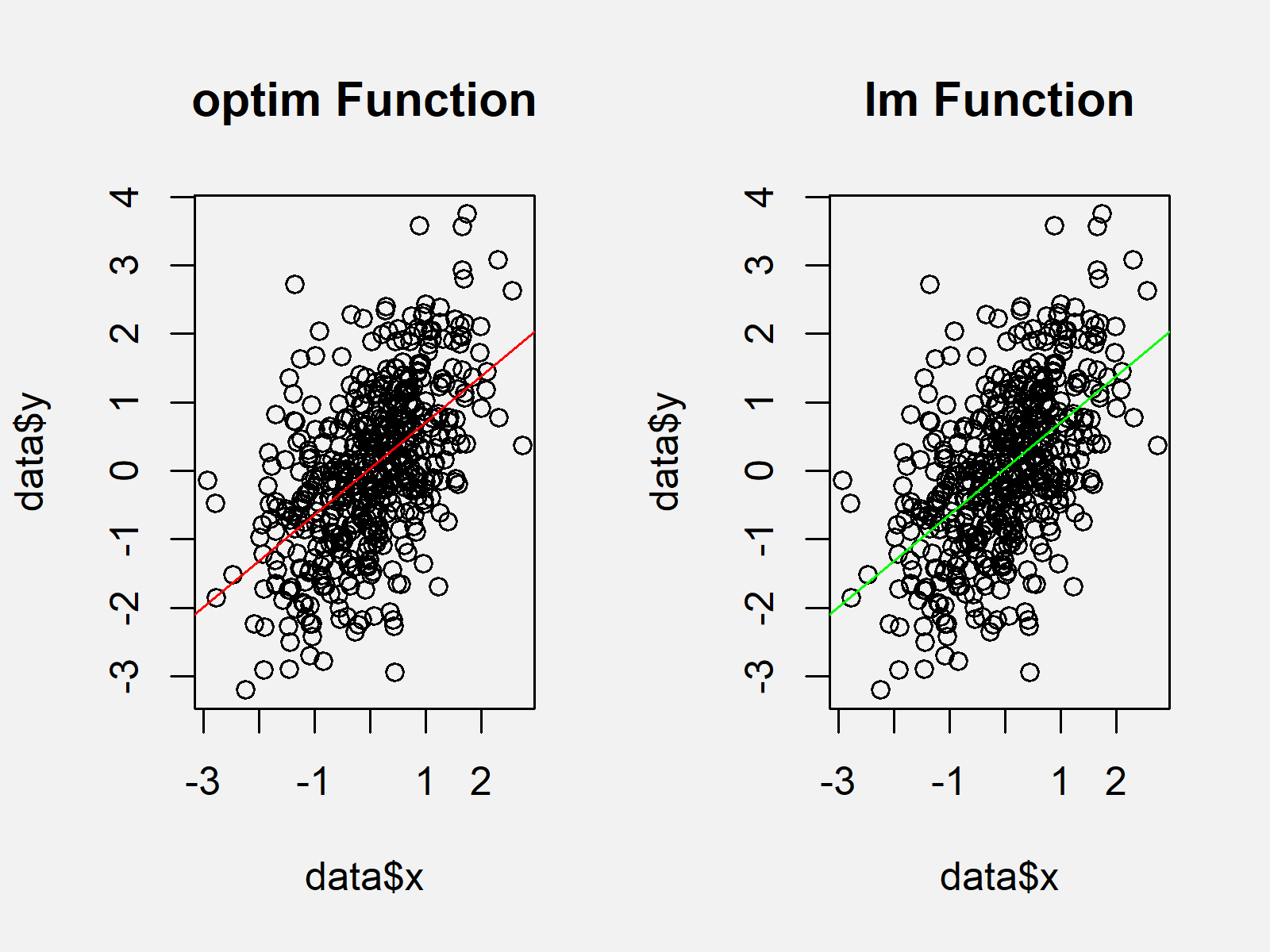# optim Function in R (Example)

On this page you’ll learn how to apply a general-purpose optimization using the optim function in the R programming language.

So without further ado, let’s dive right in:

## Creation of Example Data

The following data is used as basement for this R programming tutorial:

```set.seed(93420)                         # Creating random data
x <- rnorm(500)
y <- rnorm(500) + 0.7 * x
data <- data.frame(x, y)
#             x           y
# 1 -0.21492991 -0.06814474
# 2 -0.02217756 -0.84956484
# 3  0.55175788  0.11247758
# 4 -0.33581492 -0.86346317
# 5 -0.02489514  0.44307381
# 6 -1.44784931 -2.49701457```

The previous output of the RStudio console shows the structure of our example data: It contains two numeric variables x and y.

## Example: Applying optim Function in R

In this Example, I’ll explain how to use the optim function to minimize the residual sum of squares in the R programming language. First, we’ll manually create a function that computes the residual sum of squares. Please note that this function and the following R code is partly based on a tutorial that I found here.

```my_function <- function(data, par) {    # Own function for residual sum of squares
with(data, sum((par + par * x - y)^2))
}```

Now, we can use the optim function as shown below. The par argument specifies the initial values for the parameters to be optimized over, the fn argument specifies our function, and the data argument specifies our data frame. We are storing the output of the optim function in the data object optim_output:

```optim_output <- optim(par = c(0, 1),    # Applying optim
fn = my_function,
data = data)```

Next, we can visualize our results in a plot. For comparison, I’m going to plot our results of the optim function side-by-side with the results of a conventional linear model provided by the lm function. If we’ve done everything correctly, the output of both functions should be the same.

First, we have to specify the settings of our plot using the par function:

`par(mfrow = c(1, 2))                    # Setting plot parameters`

Now, we can draw the results of the optim function on the left side of our graphic…

```plot(data\$x,                            # Plotting results of optim function
data\$y,
main = "optim Function")
abline(optim_output\$par,
optim_output\$par,
col = "red")```

…and the results of the lm function at the right side of our graphic:

```plot(data\$x,                            # Plotting results of lm function
data\$y,
main = "lm Function")
abline(lm(y ~ x, data),
col = "green")```Figure 1 shows the output of the previous R code: Both the optim and the lm functions returned the same result. In other words, our manual optimization using the optim function worked fine.

## Video & Further Resources

Do you need further information on the R programming codes of this article? Then you might have a look at the following video of my YouTube channel. In the video, I’m showing the R programming codes of this tutorial:

Furthermore, you might read the related articles of www.statisticsglobe.com:

In summary: This page showed how to apply the optim function in the R programming language. Don’t hesitate to let me know in the comments, in case you have additional questions.

Subscribe to the Statistics Globe Newsletter